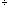Quandaries and Queries can you please tell me what the exponent 0 is (i.e. 70) Debbi; middle level; parent thank you. Hi Debbi, The convention is to make 70 = 1. The reason for this is that it makes some of the rules of arithmetic valid, even when the exponent is zero. For example 7372 = 75 and 7372 = 71 That is, to multiply two powers with the same base you add the exponents and to divide two powers with the same base you subtract the powers. But 7373 = 1 and the rule above says that 7373 = 70 Thus to make the two agree we say that 70 = 1. Penny Go to Math Central